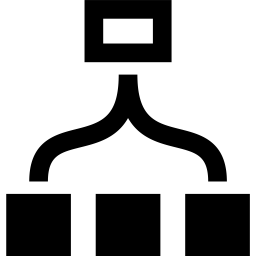# QN Index

An Index object can be given additional quantum number (QN) block structure when it is constructed. This structure cannot be changed afterward.

In addition to the QN structure, a set of tags and an Arrow direction (In,Out) can be specified when constructing an Index.

## Synopsis

// Construct an Index with QN information
// 3 blocks, total size 4+8+4=16
auto I = Index(QN({"Sz", 2}),4,
QN({"Sz", 0}),8,
QN({"Sz",-2}),4);

// Provide Arrow direction and tags
auto J = Index(QN({"T", 1}),3,
QN({"T",-1}),3,

// Make an Index with five blocks
// and total size 4+8+10+8+4=34
auto I = Index(QN(+2),4,
QN(+1),8,
QN( 0),10,
QN(-1),8,
QN(-2),4,
"I");

Print(dim(I)); //prints: dim(I) = 16

//Get number of blocks of I
Print(nblock(I)); //prints: nblock(I) = 3

Print(blocksize(I,2)); //prints: blocksize(I,2) = 8
Print(qn(I,2));    //prints: QN({"Sz",0})

//Get direction of I
Print(dir(I)); //prints: dir(I) = Out


## Index properties

• .dag()

dag(Index I) -> Index

Change the arrow direction of the Index.

• dir(Index I) -> Arrow

Return the Arrow direction of this Index.

• nblock(Index I) -> int

Return the number of QN blocks of this Index.

• qn(Index I, int j) -> QN

Return the QN of block j.

• blocksize(Index I, int j) -> int

Return the block size of block j.Back to ClassesBack to Main